# ACT Math : How to find the height of of an acute / obtuse isosceles triangle

## Example Questions

### Example Question #1 : How To Find The Height Of Of An Acute / Obtuse Isosceles Triangle

An isosceles triangle has a base of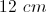and an area of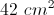. What must be the height of this triangle?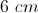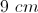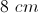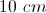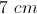Explanation: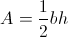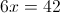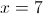### Example Question #234 : Plane Geometry

What is the height of an isosceles triangle which has a base of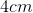and an area of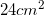?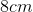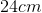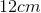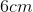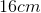Explanation:

The area of a triangle is given by the equation: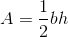In this case, we are given the area () and the base () and are asked to solve for height ().

To do this, we must plug in the given values forandwhich gives the following: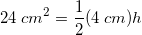We then must multiply the right side, and then divide the entire equation by 2, in order to solve for: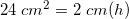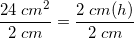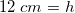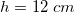Therefore, the height of the triangle is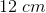.